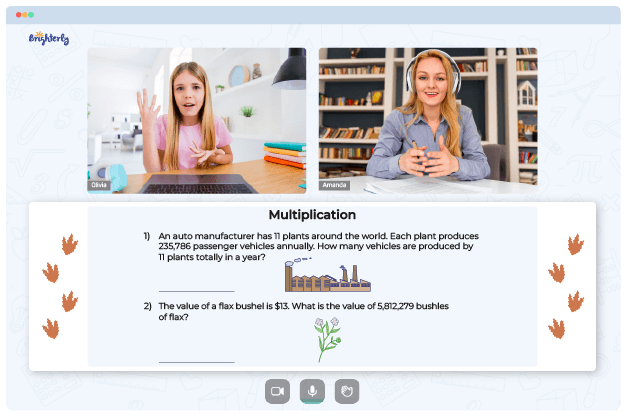# 3 Digit By 2 Digit Multiplication Worksheets

Parents who have kids in elementary school probably already know about math worksheets. Teachers use them during exams, tests, and as instructional aids.

If your kid needs help with complex multiplication like those involving three-digit numbers and two-digit ones, getting them a worksheet is wise. This article outlines some ways that 3-digit by 2-digit multiplication worksheets can help kids do better in school.

## Benefits of 3-Digit by 2-Digit Multiplication Worksheets

A digit is an integer between 0 and 9; numbers from 10 to 99 are known as two-digit numbers, and numbers ranging from 100 to 999 are known as three-digit numbers. Tutors from Brighterly can help your students multiply those numbers using a multiply 3-digit by 2-digit worksheet. These tools can be a guide to solving complex three-digit by two-digit multiplication problems.

Math for Kids

Is Your Child Struggling With Math?
1:1 Online Math TutoringKids need to practice constantly, and multiplication worksheets can give them the boost they need. Printable and easy-to-understand 3-digit by 2-digit multiplication worksheets with answers are the best of the bunch. By working on 3-digit by 2-digit multiplication word problems worksheets in PDF, kids get used to simple rules of multi-digit multiplication.

## 3-Digit by 2-Digit Multiplication Worksheets Help Teach Math in a Fun Way### 3 Digit By 2 Digit Multiplication Worksheets PDF

Multiply 3 Digit By 2-Digit Worksheet### 3 Digit By 2 Digit Multiplication Worksheets PDF

3 Digit By 2 Digit Multiplication Word Problems Worksheets PDF### 3 Digit By 2 Digit Multiplication Worksheets PDF

3 Digit By 2-Digit Multiplication Worksheets With Answers### 3 Digit By 2 Digit Multiplication Worksheets PDF

2 Digit By 3 Digit Multiplication Worksheets

2-digit by 3-digit multiplication worksheets come with a collection of questions that can help adults supervise how well kids perform when multiplying those numbers. The sheets have colorful visuals and exciting challenges that kids can use to keep themselves busy. With multiplying 2 and 3-digit numbers worksheets, you will capture your child’s attention and get them the best support system for elementary multiplication.

Helping your kid with online math lessons for 4th grade. Expert tutors inspiring kids to love math so they can excel at It.

### More Multiplication Worksheets

Troubles with Multiplication?• Does your child need extra support with mastering the concept of multiplication?
• Start lessons with an online tutor.

Is your child finding it hard to grasp of multiplication? An online tutor could be of assistance.